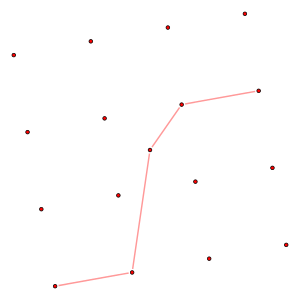# Polygonal chainA simple polygonal chainA self-intersecting polygonal chainA closed polygonal chain

In geometry, a polygonal chain is a connected series of line segments. More formally, a polygonal chain P is a curve specified by a sequence of pointscalled its vertices. The curve itself consists of the line segments connecting the consecutive vertices. A polygonal chain may also be called a polygonal curve, polygonal path, polyline, piecewise linear curve, or, in geographic information systems, a linestring or linear ring.

## Variations

A simple polygonal chain is one in which only consecutive (or the first and the last) segments intersect and only at their endpoints.

A closed polygonal chain is one in which the first vertex coincides with the last one, or, alternatively, the first and the last vertices are also connected by a line segment. A simple closed polygonal chain in the plane is the boundary of a simple polygon. Often the term "polygon" is used in the meaning of "closed polygonal chain", but in some cases it is important to draw a distinction between a polygonal area and a polygonal chain.

A polygonal chain is called monotone, if there is a straight line L such that every line perpendicular to L intersects the chain at most once. Every nontrivial monotone polygonal chain is open. In comparison, a monotone polygon is a polygon (a closed chain) that can be partitioned into exactly two monotone chains. The graphs of piecewise linear functions form monotone chains with respect to a horizontal line.A set of n=17 points has a polygonal path with 4 same-sign slopes

## Properties

Every set of at leastpoints contains a polygonal path of at leastedges in which all slopes have the same sign. This is a corollary of the Erdős–Szekeres theorem.

## Applications

Polygonal chains can often be used to approximate more complex curves. In this context, the Ramer–Douglas–Peucker algorithm can be used to find a polygonal chain with few segments that serves as an accurate approximation.

In graph drawing, polygonal chains are often used to represent the edges of graphs, in drawing styles where drawing the edges as straight line segments would cause crossings, edge-vertex collisions, or other undesired features. In this context, it is often desired to draw edges with as few segments and bends as possible, to reduce the visual clutter in the drawing; the problem of minimizing the number of bends is called bend minimization.

Polygonal chains are also a fundamental data type in computational geometry. For instance, a point location algorithm of Lee and Preparata operates by decomposing arbitrary planar subdivisions into an ordered sequence of monotone chains, in which a point location query problem may be solved by binary search; this method was later refined to give optimal time bounds for the point location problem.

With geographic information system, linestrings may represent any linear geometry, and can be described using the well-known text markup as a LineString or MultiLineString. Linear rings (or LinearRing) are closed and simple polygonal chains used to build polygon geometries.

## References

1. Gomes, Jonas; Velho, Luiz; Costa Sousa, Mario (2012), Computer Graphics: Theory and Practice, CRC Press, p. 186, ISBN 9781568815800.
2. Cheney, Ward (2001), Analysis for Applied Mathematics, Graduate Texts in Mathematics, 208, Springer, p. 13, ISBN 9780387952796.
3. Boissonnat, Jean-Daniel; Teillaud, Monique (2006), Effective Computational Geometry for Curves and Surfaces, Springer, p. 34, ISBN 9783540332596.
4. Open Geospatial Consortium (2011-05-28), Herring, John R., ed., OpenGIS® Implementation Standard for Geographic information - Simple feature access - Part 1: Common architecture, 1.2.1, Open Geospatial Consortium, retrieved 2016-01-15
5. Mehlhorn, Kurt; Näher, Stefan (1999), LEDA: A Platform for Combinatorial and Geometric Computing, Cambridge University Press, p. 758, ISBN 9780521563291.
6. O'Rourke, Joseph (1998), Computational Geometry in C, Cambridge Tracts in Theoretical Computer Science, Cambridge University Press, p. 45, ISBN 9780521649766.
7. Ramer, Urs (1972), "An iterative procedure for the polygonal approximation of plane curves", Computer Graphics and Image Processing, 1 (3): 244–256, doi:10.1016/S0146-664X(72)80017-0.
8. Douglas, David; Peucker, Thomas (1973), "Algorithms for the reduction of the number of points required to represent a digitized line or its caricature", The Canadian Cartographer, 10 (2): 112–122, doi:10.3138/FM57-6770-U75U-7727.
9. Tamassia, Roberto (1987), "On embedding a graph in the grid with the minimum number of bends", SIAM Journal on Computing, 16 (3): 421–444, doi:10.1137/0216030.
10. Edelsbrunner, Herbert; Guibas, Leonidas J.; Stolfi, Jorge (1986), "Optimal point location in a monotone subdivision", SIAM Journal on Computing, 15 (2): 317–340, doi:10.1137/0215023.